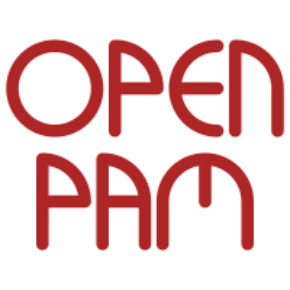OpenPAM
/
OpenPAM
You cannot select more than 25 topics Topics must start with a letter or number, can include dashes ('-') and can be up to 35 characters long.

#### 33 lines 725 B Makefile Raw Permalink Blame History

 ```if WITH_TEST ``` ``` ``` ```AM_CPPFLAGS = -I\$(top_srcdir)/include -I\$(top_srcdir)/lib/libpam \ ``` ``` \$(CRYB_TEST_CFLAGS) ``` ``` ``` ```AM_TESTS_ENVIRONMENT = \ ``` ``` PAM_RETURN_SO=\$(abs_top_builddir)/modules/pam_return/.libs/pam_return.so ``` ``` ``` ```# tests ``` ```TESTS = ``` ```TESTS += t_openpam_ctype ``` ```TESTS += t_openpam_dispatch ``` ```TESTS += t_openpam_readword ``` ```TESTS += t_openpam_readlinev ``` ```TESTS += t_openpam_straddch ``` ```TESTS += t_pam_env ``` ```check_PROGRAMS = \$(TESTS) ``` ``` ``` ```# libt - common support code ``` ```check_LIBRARIES = libt.a ``` ```libt_a_SOURCES = t_pam_conv.c t_pam_err.c ``` ```noinst_HEADERS = t_pam_conv.h t_pam_err.h ``` ``` ``` ```# link with libpam and test framework ``` ```LDADD = \$(CRYB_TEST_LIBS) libt.a ``` ```if WITH_SYSTEM_LIBPAM ``` ```LDADD += \$(SYSTEM_LIBPAM) ``` ```else ``` ```LDADD += \$(top_builddir)/lib/libpam/libpam.la ``` ```endif ``` ``` ``` ```endif ```